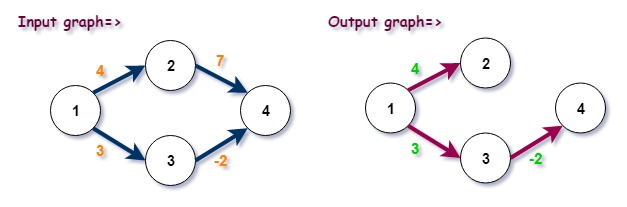# Bellman Ford algorithm in C++

In this tutorial, we will learn about the bellman ford algorithm and its implementation in C++.

Bellman-Ford algorithm: is a single source shortest path algorithm that is used to find out the shortest paths from a single source vertex to all of the other vertices in a weighted directed graph. It can work with graphs with negative edge weights. On the other hand, Dijkstra’s algorithm cannot work with graphs with negative edge weights. It is slower than Dijkstra’s algorithm. It helps to detect the negative cycle in the graph.

Negative Cycle: is a cycle with weights that sum to a negative number.

Steps of Bellman-Ford algorithm:

1. Initialize distances from the source to all vertices as infinite and distance to the source itself as 0.
2. for each edge a->b of the graph
If dis[b] > dis[a] + weight of edge (a->b) then
dis[b] = dis[a] + weight of edge (a->b)
3. repeat step 2 for nv-1 times (nv is no. of vertices).
4. If dis[b] > dis[a] + weight of edge (a->b) then
report a negative cycle in the graph.## Program to implement Bellman ford algorithm in C++

```#include<iostream>
#define MAX 10
using namespace std;

typedef struct edge
{
int src;
int dest;
int wt;
}edge;

void bellman_ford(int nv,edge e[],int src_graph,int ne)
{
int u,v,weight,i,j=0;
int dis[MAX];

/* initializing array 'dis' with 999. 999 denotes infinite distance */
for(i=0;i<nv;i++)
{
dis[i]=999;
}

/* distance of source vertex from source vertex is o */
dis[src_graph]=0;

/* relaxing all the edges nv - 1 times */
for(i=0;i<nv-1;i++)
{
for(j=0;j<ne;j++)
{
u=e[j].src;
v=e[j].dest;
weight=e[j].wt;

if(dis[u]!=999 && dis[u]+weight < dis[v])
{
dis[v]=dis[u]+weight;
}
}

}

/* checking if negative cycle is present */
for(j=0;j<ne;j++)
{
u=e[j].src;
v=e[j].dest;
weight=e[j].wt;

if(dis[u]+weight < dis[v])
{
cout<<"\n\nNEGATIVE CYCLE PRESENT..!!\n";
return;
}
}

cout<<"\nVertex"<<"  Distance from source";
for(i=1;i<=nv;i++)
{
cout<<"\n"<<i<<"\t"<<dis[i];
}

}

int main()
{
int nv,ne,src_graph;
edge e[MAX];

cout<<"Enter the number of vertices: ";
cin>>nv;

/* if you enter no of vertices: 5 then vertices will be 1,2,3,4,5. so while giving input enter source and destination vertex accordingly */
printf("Enter the source vertex of the graph: ");
cin>>src_graph;

cout<<"\nEnter no. of edges: ";
cin>>ne;

for(int i=0;i<ne;i++)
{
cout<<"\nFor edge "<<i+1<<"=>";
cout<<"\nEnter source vertex :";
cin>>e[i].src;
cout<<"Enter destination vertex :";
cin>>e[i].dest;
cout<<"Enter weight :";
cin>>e[i].wt;
}

bellman_ford(nv,e,src_graph,ne);

return 0;
}```

Input/Output:

```Enter the number of vertices: 4
Enter the source vertex of the graph: 1

Enter no. of edges: 4

For edge 1=>
Enter source vertex :1
Enter destination vertex :2
Enter weight :4

For edge 2=>
Enter source vertex :1
Enter destination vertex :3
Enter weight :3

For edge 3=>
Enter source vertex :2
Enter destination vertex :4
Enter weight :7

For edge 4=>
Enter source vertex :3
Enter destination vertex :4
Enter weight :-2

Vertex  Distance from source
1       0
2       4
3       3
4       1```

#### Time Complexity

O(V*E), where V is no. of vertices and E is no. of edges in the graph.